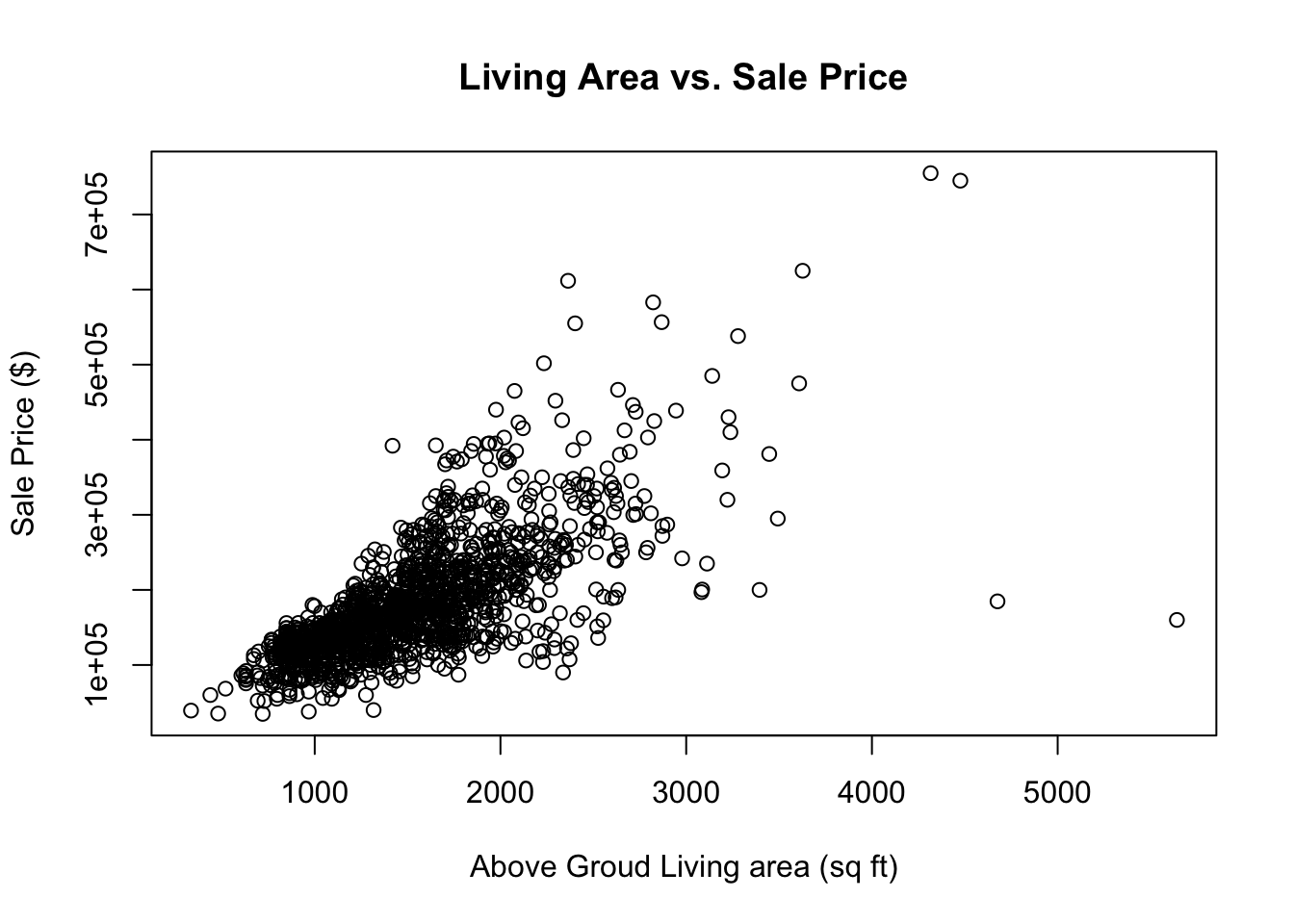Problem 1. Using R, generate a random variable X that has 10,000 random uniform numbers from 1 to N, where N can be any number of your choosing greater than or equal to 6. Then generate a random variable Y that has 10,000 random normal numbers with a mean of \$ = = (N+1)/2\$

``````# generate random variables
set.seed(123)
N <- 8
n <- 10000
mu <- (N+1) /2
X <- runif(n, min = 1, max = N)
Y <- rnorm(n, mean = mu)
x <- median(X)
y <- quantile(Y, 0.25)
XY <- data.frame(cbind(X,Y))``````

Calculate as a minimum the below probabilities a through c. Assume the small letter “x” is estimated as the median of the X variable, and the small letter “y” is estimated as the 1st quartile of the Y variable. Interpret the meaning of all probabilities.

1. P(X>x | X>y) b. P(X>x, Y>y) c. P(X<x | X>y)

The conditional probability - Probability of A given B occurs

\$ P(A | B) = = = \$

``````# a. P(X>x | X>y)
# P(X>4.46 | X > 3.83)

pY <- sum(X>y) /n
pX_pY <- sum(X>x & X>y) / n
pAB <- round(pX_pY/pY, 3)
print(paste0("P(X>x | X>y) = ",pAB))``````
``##  "P(X>x | X>y) = 0.837"``
``````# b. P(X>x, Y>y)
pXandpY <- round(sum(X>x & Y>y)/n, 3)
print(paste0("P(X>x, Y>y) = ", pXandpY))``````
``##  "P(X>x, Y>y) = 0.376"``
``````# c.  P(X<x | X>y)
pY2 <- sum(X>y) /n
pX_pY2 <- sum(X<x & X>y) / n
pAB2 <- round(pX_pY2/pY2, 3)
print(paste0("P(X<x | X>y) = ",pAB2))``````
``##  "P(X<x | X>y) = 0.163"``

Investigate whether P(X>x and Y>y)=P(X>x)P(Y>y) by building a table and evaluating the marginal and joint probabilities.

``````# build probability table
a <- sum(X>x & Y>y) /n
b <- sum(X<x & Y>y) /n
t1 <- sum(a,b)
c <- sum(X>x & Y<y) /n
d <- sum(Y<y & X<x) /n
t2 <- sum(c, d)
t3 <- sum(a, c)
t4 <- sum(b, d)
t5 <- sum(t1, t2)
probMatrix <- matrix(c(a, b, t1, c, d, t2, t3, t4, t5), nrow = 3, byrow = T)
colnames(probMatrix) <- c("X>x", "X<x", "total")
row.names(probMatrix) <- c("Y>y", "Y<y", "total")
probMatrix <- as.table(probMatrix)
probMatrix``````
``````##          X>x    X<x  total
## Y>y   0.3756 0.3744 0.7500
## Y<y   0.1244 0.1256 0.2500
## total 0.5000 0.5000 1.0000``````
``````# evaluate if P(X>x and Y>y)=P(X>x)P(Y>y)

probMatrix[1,1]/probMatrix[1,3] * (probMatrix[1,1]/probMatrix[3,1]) # P(X>x)P(Y>y)``````
``##  0.376201``
``probMatrix[1,1] # P(X>x and Y>y)``
``##  0.3756``
``# Both probabilities are aprox.equal, which proves P(X>x and Y>y)=P(X>x)P(Y>y)``

Check to see if independence holds by using Fisher’s Exact Test and the Chi Square Test. What is the difference between the two? Which is most appropriate? Fisher’s exact test examines the significance of associationg between classifications for small sample sizes; Chi Square test can be used when samples are large.

``fisher.test(table(X>x, Y>y))``
``````##
##  Fisher's Exact Test for Count Data
##
## data:  table(X > x, Y > y)
## p-value = 0.7995
## alternative hypothesis: true odds ratio is not equal to 1
## 95 percent confidence interval:
##  0.9242273 1.1100187
## sample estimates:
## odds ratio
##   1.012883``````
``chisq.test(table(X>x, Y>y))``
``````##
##  Pearson's Chi-squared test with Yates' continuity correction
##
## data:  table(X > x, Y > y)
## X-squared = 0.064533, df = 1, p-value = 0.7995``````

p-values from both test are larger than 0.05, we do not reject the null hypothese that the two variables are independent of each other. Our sample size is relatively large n=10000, therefore the Chi squared test is more appropriate.

Problem 2.

``library(Hmisc)``
``## Loading required package: lattice``
``## Loading required package: survival``
``## Loading required package: Formula``
``## Loading required package: ggplot2``
``````##
## Attaching package: 'Hmisc'``````
``````## The following objects are masked from 'package:base':
##
##     format.pval, units``````
``library(corrplot)``
``## corrplot 0.84 loaded``
``````library(MASS)
library(ggplot2)``````

Provide univariate descriptive statistics and appropriate plots for the training data set.

``````# load dataset

# plot linear regression model
plot(train\$GrLivArea, train\$SalePrice, main = "Living Area vs. Sale Price", xlab = "Above Groud Living area (sq ft)", ylab = "Sale Price (\$)")````````````train.lm <- lm(train\$SalePrice ~ train\$GrLivArea)
summary(train.lm)``````
``````##
## Call:
## lm(formula = train\$SalePrice ~ train\$GrLivArea)
##
## Residuals:
##     Min      1Q  Median      3Q     Max
## -462999  -29800   -1124   21957  339832
##
## Coefficients:
##                  Estimate Std. Error t value Pr(>|t|)
## (Intercept)     18569.026   4480.755   4.144 3.61e-05 ***
## train\$GrLivArea   107.130      2.794  38.348  < 2e-16 ***
## ---
## Signif. codes:  0 '***' 0.001 '**' 0.01 '*' 0.05 '.' 0.1 ' ' 1
##
## Residual standard error: 56070 on 1458 degrees of freedom
## Multiple R-squared:  0.5021, Adjusted R-squared:  0.5018
## F-statistic:  1471 on 1 and 1458 DF,  p-value: < 2.2e-16``````
``````# residual analysis to evaluate model quality
plot(fitted(train.lm, resid(train.lm)))``````